### Home > A2C > Chapter 4 > Lesson 4.1.4 > Problem4-52

4-52.
1. Draw accurate graphs of y = 2x + 5, y = 2x2 + 5, and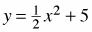on the same set of axes. Label the intercepts. 4-52 HW eTool (Desmos). Homework Help ✎

1. In the equation y = 2x + 5, what does the 2 tell you about the graph?

2. Is the 2 in y = 2x2 + 5 also the slope? Explain.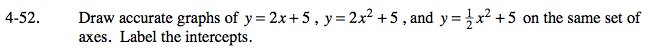Use the eTool below to view the graphs.
Click the link at right for the full version of the eTool: A2C 4-52 HW eTool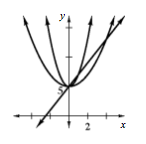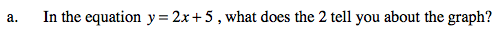The 2 is the slope of the line.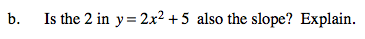Does the graph show a constant rate of change from point to point?